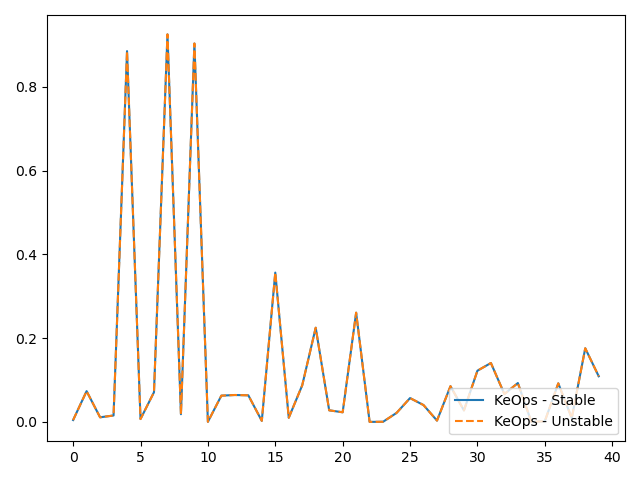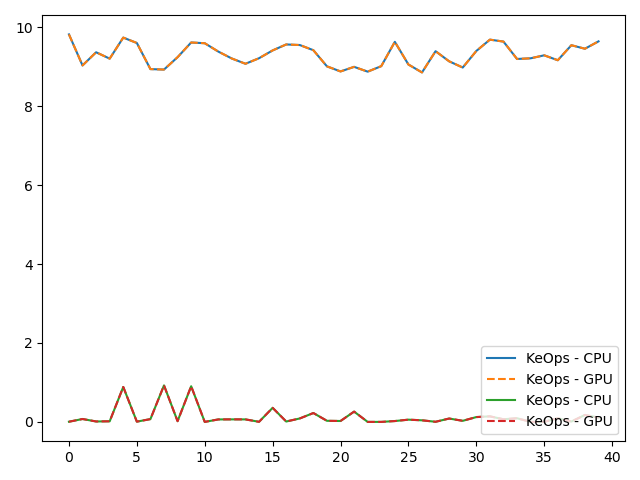# LogSumExp reduction

Let’s compute the (3000,1) tensor $$c$$ whose entries $$c_i$$ are given by:

$c_i = \log \left[ \sum_j \exp\left( (p-a_j)^2 \exp(x_i+y_j) \right) \right]$

where

• $$x$$ is a (3000,1) tensor, with entries $$x_i$$.

• $$y$$ is a (5000,1) tensor, with entries $$y_j$$.

• $$a$$ is a (5000,1) tensor, with entries $$a_j$$.

• $$p$$ is a scalar, encoded as a vector of size (1,).

## Setup

Standard imports:

import time

import torch
from matplotlib import pyplot as plt

from pykeops.torch import Genred


Declare random inputs:

M = 3000
N = 5000

dtype = "float32"  # Could be 'float32' or 'float64'
torchtype = torch.float32 if dtype == "float32" else torch.float64

x = torch.rand(M, 1, dtype=torchtype)
y = torch.rand(N, 1, dtype=torchtype, requires_grad=True)
a = torch.rand(N, 1, dtype=torchtype)
p = torch.rand(1, dtype=torchtype)


## Define a custom formula

formula = "Square(p-a)*Exp(x+y)"
variables = [
"x = Vi(1)",  # First arg   : i-variable, of size 1 (scalar)
"y = Vj(1)",  # Second arg  : j-variable, of size 1 (scalar)
"a = Vj(1)",  # Third arg   : j-variable, of size 1 (scalar)
"p = Pm(1)",
]  # Fourth  arg : Parameter,  of size 1 (scalar)

start = time.time()


Our log-sum-exp reduction is performed over the index $$j$$, i.e. on the axis 1 of the kernel matrix. The output c is an $$x$$-variable indexed by $$i$$.

my_routine = Genred(formula, variables, reduction_op="LogSumExp", axis=1)
c = my_routine(x, y, a, p, backend="CPU")

# N.B.: By specifying backend='CPU', we can make sure that the result is computed using a simple C++ for loop.
print(
"Time to compute the convolution operation on the cpu: ",
round(time.time() - start, 5),
"s",
end=" ",
)

Time to compute the convolution operation on the cpu:  0.04878 s


We compare with the unstable, naive computation “Log of Sum of Exp”:

my_routine2 = Genred(
"Exp(" + formula + ")", variables, reduction_op="Sum", axis=1, dtype=dtype
)
c2 = torch.log(my_routine2(x, y, a, p, backend="CPU"))
print("(relative error: ", ((c2 - c).norm() / c.norm()).item(), ")")

# Plot the results next to each other:
plt.plot(c.detach().cpu().numpy()[:40], "-", label="KeOps - Stable")
plt.plot(c2.detach().cpu().numpy()[:40], "--", label="KeOps - Unstable")
plt.legend(loc="lower right")
plt.tight_layout()
plt.show()[pyKeOps] Warning : keyword argument dtype in Genred is deprecated ; argument is ignored.
(relative error:  4.9228258802713754e-08 )


Now, let’s compute the gradient of $$c$$ with respect to $$y$$. Since $$c$$ is not scalar valued, its “gradient” $$\partial c$$ should be understood as the adjoint of the differential operator, i.e. as the linear operator that:

• takes as input a new tensor $$e$$ with the shape of $$c$$

• outputs a tensor $$g$$ with the shape of $$y$$

such that for all variation $$\delta y$$ of $$y$$ we have:

$\langle \text{d} c . \delta y , e \rangle = \langle g , \delta y \rangle = \langle \delta y , \partial c . e \rangle$

Backpropagation is all about computing the tensor $$g=\partial c . e$$ efficiently, for arbitrary values of $$e$$:

# Declare a new tensor of shape (M,1) used as the input of the gradient operator.
# It can be understood as a "gradient with respect to the output c"
# and is thus called "grad_output" in the documentation of PyTorch.
e = torch.rand_like(c)
start = time.time()
# PyTorch remark : grad(c, y, e) alone outputs a length 1 tuple, hence the need for  at the end.

print(
"Time to compute gradient of convolution operation on the cpu: ",
round(time.time() - start, 5),
"s",
end=" ",
)

Time to compute gradient of convolution operation on the cpu:  0.08137 s


We compare with gradient of Log of Sum of Exp:

g2 = grad(c2, y, e)
print("(relative error: ", ((g2 - g).norm() / g.norm()).item(), ")")

# Plot the results next to each other:
plt.plot(g.detach().cpu().numpy()[:40], "-", label="KeOps - Stable")
plt.plot(g2.detach().cpu().numpy()[:40], "--", label="KeOps - Unstable")
plt.legend(loc="lower right")
plt.tight_layout()
plt.show()(relative error:  6.416499331862724e-08 )


## Same operations performed on the Gpu

Of course, this will only work if you own a Gpu…

if torch.cuda.is_available():
# first transfer data on gpu
pc, ac, xc, yc, ec = p.cuda(), a.cuda(), x.cuda(), y.cuda(), e.cuda()
# then call the operations
start = time.time()
c3 = my_routine(xc, yc, ac, pc, backend="GPU")
print(
"Time to compute convolution operation on the gpu:",
round(time.time() - start, 5),
"s ",
end="",
)
print("(relative error:", float(torch.abs((c2 - c3.cpu()) / c2).mean()), ")")
start = time.time()
print(
"Time to compute gradient of convolution operation on the gpu:",
round(time.time() - start, 5),
"s ",
end="",
)
print("(relative error:", float(torch.abs((g2 - g3.cpu()) / g2).mean()), ")")

# Plot the results next to each other:
plt.plot(c.detach().cpu().numpy()[:40], "-", label="KeOps - CPU")
plt.plot(c3.detach().cpu().numpy()[:40], "--", label="KeOps - GPU")
plt.legend(loc="lower right")
plt.tight_layout()
plt.show()

# Plot the results next to each other:
plt.plot(g.detach().cpu().numpy()[:40], "-", label="KeOps - CPU")
plt.plot(g3.detach().cpu().numpy()[:40], "--", label="KeOps - GPU")
plt.legend(loc="lower right")
plt.tight_layout()
plt.show()Time to compute convolution operation on the gpu: 0.01607 s (relative error: 2.072763649607623e-08 )
Time to compute gradient of convolution operation on the gpu: 0.014 s (relative error: 1.3177152879961795e-07 )


Total running time of the script: ( 0 minutes 0.610 seconds)

Gallery generated by Sphinx-Gallery